## Calculate context effects in binomial GLM analysis

#### Suppose your data look like this…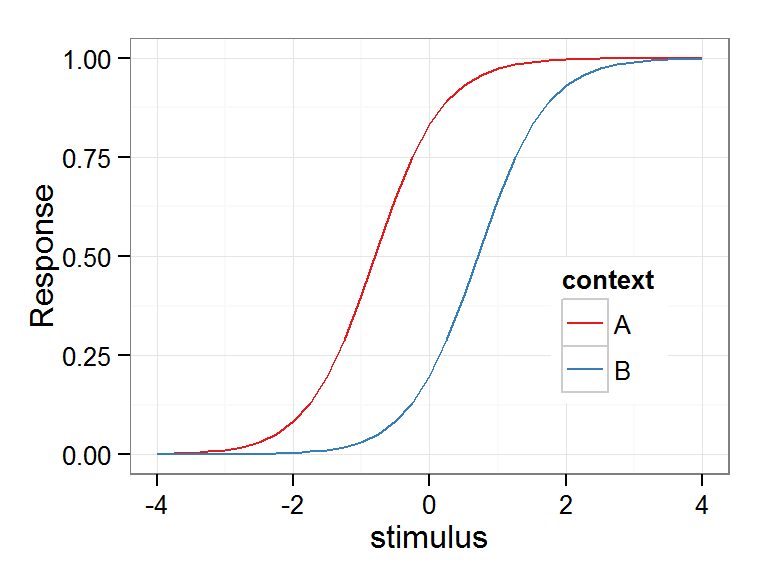… and you want to quantify the distance between the two curves in terms of horizontal displacement.

You can think of this as the difference between the points at which the curves cross the 0.5 mark on the y-axis.

(this is a simple case where the slope of each curve is the same; when slopes are different, you might want to consider whether the 50% point is really the only thing you’re interested in)

This situation is commonly known in the speech perception literature as a context effect. For example, in the context of a high-frequency precursor sound, a target stimulus will sound lower, and in the context of a low-frequency precursor sound, the target stimulus will sound higher in comparison.
In short, the perception of a stimulus depends on its local context.
There are numerous papers that look at speech context effects… but… this is my website, so I’m linking my paper with Ariane Rhone, Monita Chatterjee and Bill Idsardi.

### In this post, we explore the quantification of the effect of the context on the perception of a stimulus.

Let’s begin.
First, let’s load some packages and make up some data

library(lme4)
library(ggplot2)
# remove all objects
rm(list=ls())
x <- seq(-4, 4, 0.25)
y <- 1/(1+exp(-2*x-2.6))
z <- 1/(1+exp(-2*x+2.4))

fake.data <- data.frame(stimulus = rep(x,2),
context = c(rep("A", length(x)),
rep("B", length(x))),
Response = c(y,z))

Plot it out

p <- ggplot(fake.data, aes(x=stimulus, y=Response, color=context))+
geom_line()+
theme_bw()+
scale_color_brewer(palette = "Set1")+
theme(legend.position=c(0.8, 0.33))
p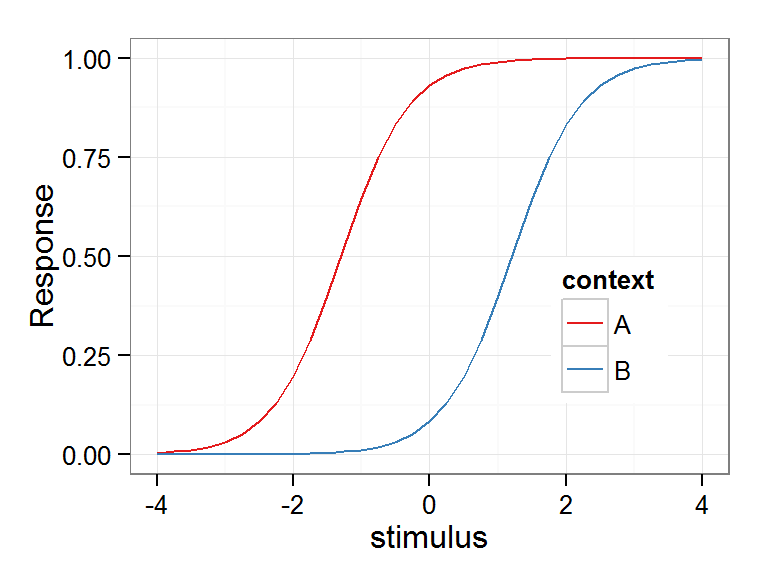Those were idealized curves.

#### Let’s make some binomial data

Real binomial data is not a bunch of proportions, but a collection of a bunch of 1s and 0s (or hits and misses).

To generate that, I’m going to use some plyr magic.
ddply is a function that takes as its first argument a data frame. The second argument .() is a list of unevaluated variables by which the data fame will be subsetted. So when you see .(stimulus, context), it organizes the data into every possible combination of all the levels of stimulus and all the levels of context.
For each of those subsets, a function is applied.
Here, the function needs to create a table of binomial observations that would produce the proportion seen in the data plotted above.
For example, if your proportion is 0.9, you’d need 9 hits and 1 miss, or 90 hits and 10 misses.
If the number of observations doesn’t cleanly multiply by the proportion, it will be rounded to the nearest natural number.
we’ll generate 50 observations for fun.

num.obs <- 50
fake.data.binomial <- ddply(fake.data, .(stimulus, context), .fun=function(df){
hits <- round(df$Response * num.obs, digits=0) misses <- num.obs - hits return(data.frame(Response = c(rep(x=1,times = hits), rep(x=0, times = misses)))) } ) ### Generalized linear model. Predict Response by stimulus value, Context and their interaction. Use binomial (logit) linking function. mod <- glm(Response ~ stimulus*context, data = fake.data.binomial, family=binomial) Look at the summary of the model summary(mod) ## ## Call: ## glm(formula = Response ~ stimulus * context, family = binomial, ## data = fake.data.binomial) ## ## Deviance Residuals: ## Min 1Q Median 3Q Max ## -2.9245 -0.1907 0.0059 0.1673 3.0085 ## ## Coefficients: ## Estimate Std. Error z value Pr(>|z|) ## (Intercept) 2.71510 0.18480 14.69 <2e-16 *** ## stimulus 2.06244 0.11810 17.46 <2e-16 *** ## contextB -5.16458 0.25256 -20.45 <2e-16 *** ## stimulus:contextB 0.00272 0.16685 0.02 0.99 ## --- ## Signif. codes: 0 '***' 0.001 '**' 0.01 '*' 0.05 '.' 0.1 ' ' 1 ## ## (Dispersion parameter for binomial family taken to be 1) ## ## Null deviance: 4574 on 3299 degrees of freedom ## Residual deviance: 1267 on 3296 degrees of freedom ## AIC: 1275 ## ## Number of Fisher Scoring iterations: 7 Notice how the interaction of stimulus (slope) with context is extremely low, with a p value of virtually 1. That’s because we explicitly coded the slopes to be the same :) I kept the interaction in this code in case you want to play around with different slopes. Okay, play time is over - now let’s get the coefficients from the model, assign them to an object called ‘coefs’ coefs <- summary(mod)$coefficients

Get the specific coefficients, and name them to be used in formulas later

intercept.def <- coefs["(Intercept)","Estimate"]
slope.stimulus.def <- coefs["stimulus","Estimate"]
slope.stimulus.adj.context.B <- coefs["stimulus:contextB","Estimate"]

#### Re-name the coefficients using plain-language names

based on how we set up the input variables.
Yes, this is inefficient, but the point is to demonstrate how to properly interpret the coefficients.
For example, the intercept for context B is the default intercept plus the effect of context B, which I have named as intercept.adj.context.B.

Intercept.A <- intercept.def
slope.stimulus.A <- slope.stimulus.def
slope.stimulus.B <- slope.stimulus.A + slope.stimulus.adj.context.B

Okay, now predict some data using those model parameters

stimulus.levels <- sort(unique(fake.data\$stimulus))
predicted.A <- 1/(1+exp(-(Intercept.A +
slope.stimulus.A*(stimulus.levels))))
predicted.B <- 1/(1+exp(-(Intercept.B +
slope.stimulus.B*(stimulus.levels))))

Put it in a data frame so it can be plotted

predicted.data <- data.frame(stimulus=rep(stimulus.levels,2),
context=c(rep("A", length(stimulus.levels)),
rep("B", length(stimulus.levels))),
Response=c(predicted.A, predicted.B))

First plot the original ‘data’

data.plot <- ggplot(fake.data.binomial, aes(x=stimulus, y=Response, color=context))+
stat_summary(fun.data=mean_se, geom="pointrange")+
scale_color_brewer(palette="Set1")+theme_bw()
print(data.plot)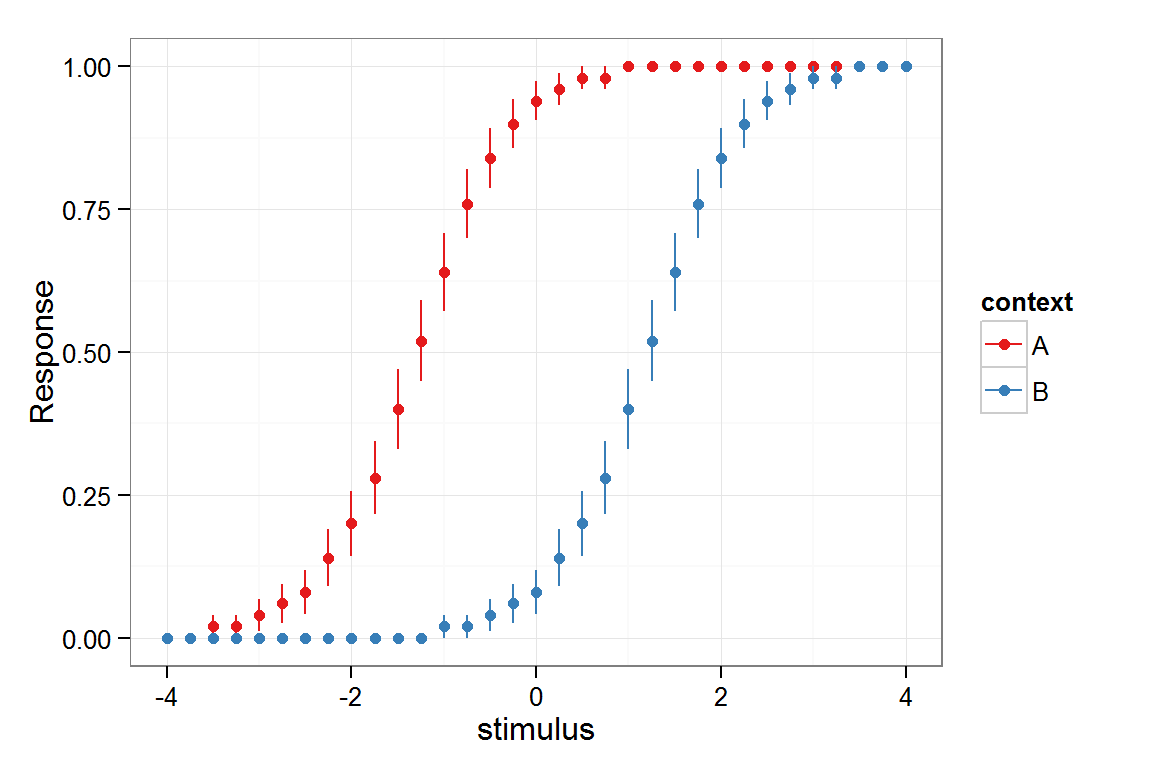Now overlay lines that represent the predicted data from the model

plot.predict <-data.plot+geom_line(data=predicted.data, stat="summary", fun.y="mean")
print(plot.predict)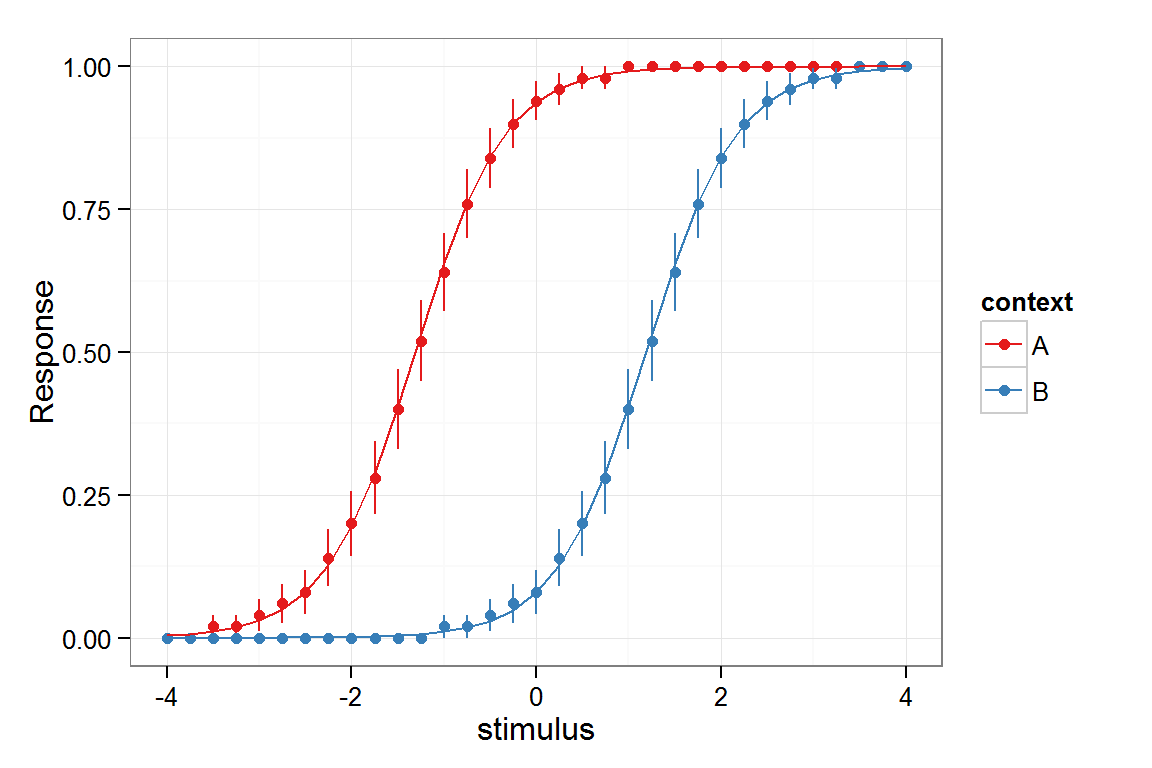Good match. Model has ben verified.
Just for fun, here’s a kludge that I use to indicate the difference between data and model predictions:

plot.predict.2 <- ggplot(fake.data.binomial,
aes(x=stimulus, y=Response, color=context))+
# data summary
stat_summary(fun.data=mean_se, geom="pointrange")+
# model prediction
geom_line(data=predicted.data, stat="summary", fun.y="mean")+
# line outside plot range, just for creating the legend
geom_line(data=subset(fake.data.binomial, context=="A"),
aes(y=-1, linetype=context))+
# ensure that the plot isn't extended to the fake line
ylim(c(0,1))+
scale_color_brewer(palette="Set1", name="Data",
labels=c("Context A", "Context B"))+
theme_bw()+
# linetype legend indicates model fit
scale_linetype_discrete(name="", labels="Model fit")+
# put linetype legend at the bottom of the list
guides(color=guide_legend(order = 1),
linetype=guide_legend(order = 2))
plot.predict.2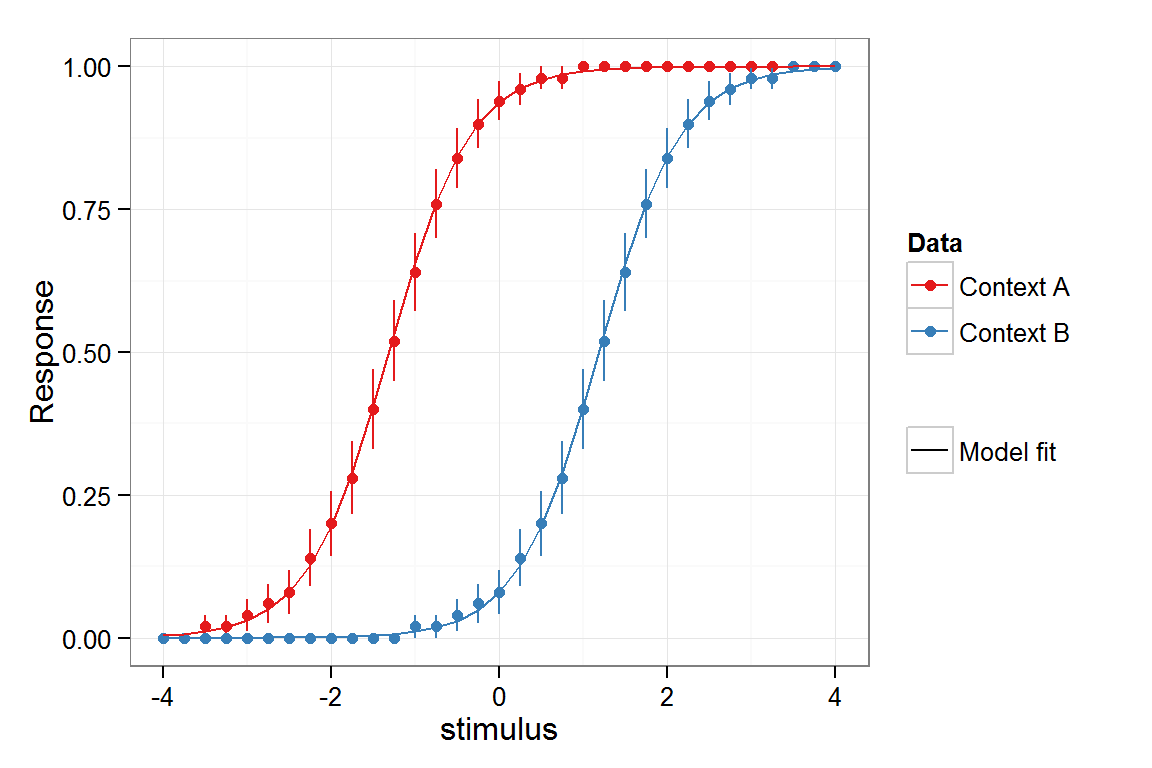### Calculating the crossover boundaries

Now, let’s calculate 50% crossover boundary for each condition.
You’re basically looking for when the formula gives us a proportion of 0.5.

In other words… we want to find out when
1/(1+exp(-(Int + coef$$\times$$(variable.level)))) is 0.5

We know the intercept and coefficient from the model summary, so we have to figure out the variable.level that fits this equation.

###### first, multiply both sides by 2

2/(1+exp(-(Int + coef$$\times$$(variable.level)))) = 1

###### multiply both sides by demonimator

2 = 1+exp(-(Int + coef$$\times$$(variable.level)))

###### subtract 1 from each side

1 = exp(-(Int + coef$$\times$$(variable.level)))

###### take the natural log of each side

(for exp(x) = 1, x is 0)
-(Int + coef$$\times$$(variable.level)) = 0

###### multiply ech side by -1

Int + coef$$\times$$variable.level = 0

###### subtract Int from each side

coef$$\times$$variable.level = -Int

###### divide each side by coef

variable.level = -Int/coef

### -Intercept / coefficient

##### for that variable, for that condition.

Remember that inefficient re-naming I did for those model coefficients?
Turns out that was a good idea. Now I can clearly see what needs to be done to calculate the category boundaries.

stimulus.level.A.50 <- as.numeric(-Intercept.A /slope.stimulus.A)
stimulus.level.B.50 <- as.numeric(-Intercept.B /slope.stimulus.B)

The “context effect” is the 50% stimulus level from context.B minus that from context.A

context.effect <- stimulus.level.B.50 - stimulus.level.A.50
context.effect
##  2.503

## Visualize the context effect

Put it on the plot

plot.boundaries <- plot.predict +
geom_vline(xintercept=stimulus.level.A.50, linetype="dashed")+
geom_vline(xintercept=stimulus.level.B.50, linetype="dashed")+
geom_segment(aes(x=stimulus.level.A.50, xend=stimulus.level.B.50,
y=0.5, yend=0.5), arrow=arrow(ends="both"),
color="#747474")+
annotate("text", x=mean(c(stimulus.level.A.50,stimulus.level.B.50)),
y=0.55, label="Context Effect", hjust=0.5)
plot.boundaries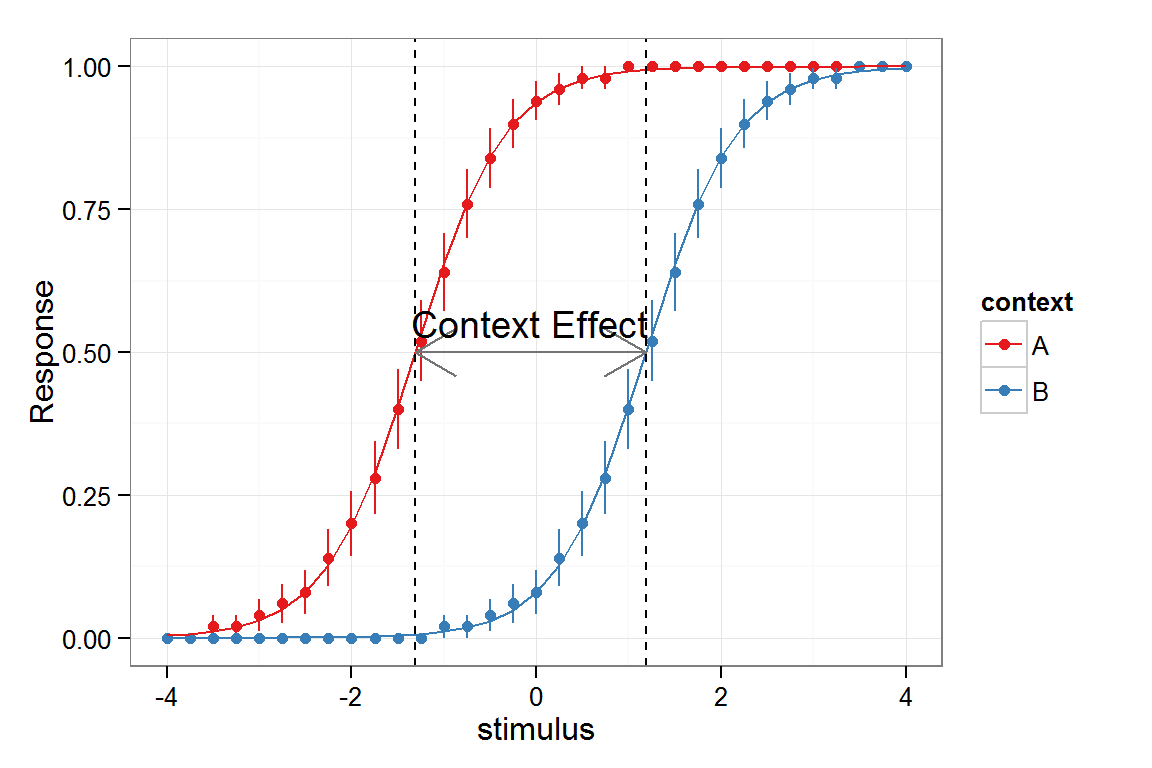Now, what was the context effect when translated to our original input range?

context.effect
##  2.503

The 50% crossover point for context B is 2.503 stimulus units away from that for context A.
The figure verifies that. Success!

This is an R Markdown document. Markdown is a simple formatting syntax for authoring HTML, PDF, and MS Word documents. For more details on using R Markdown see http://rmarkdown.rstudio.com.